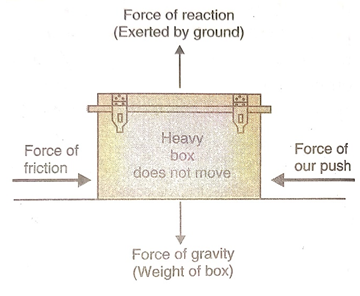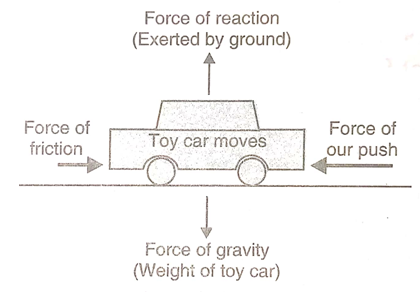# Balanced and Unbalanced Forces

Everything around us performs motion and to do this, it needs some kind of force. These forces exist in various forms. They can be pushing, pulling, lifting, stretching, twisting or pressing forces and every moving body around us is doing motion with the help of these forces. These forces can be of two types – balanced and unbalanced forces.

Let us start looking at this classification type in more detail one by one.

### Balanced forces

If the resultant of all the forces acting on the body is zero, the forces are called balanced forces.

A body under the action of balanced forces does not change its position of rest or of uniform motion with respect to time and it appears as if no fore is acting on it. Let us look at some examples.

Suppose a heavy box is lying on the ground. Let us push this box with our hands. We find that the box does not move and remains in its state of rest though as many as four forces are acting on it. The four forces acting on the box are:• Force of push
• Force of friction which opposes the push and does not allow the box to move
• Force of gravity which pulls the box downwards
• Force of reaction exerted by the ground on the box upwards which balances the force of gravity.

Now, though the box is at rest, four forces are acting on it. Since the box does not move at all, we conclude that the resultant of all the forces acting on it is zero. The box, therefore, behaves as if no force is acting on it. The forces acting on this stationary box are an example of balanced forces.

It is to be noted that the force of our push on the box is balanced by the force of friction and the force of gravity is balanced by the force of reaction on the ground.

If a number of balanced forces act on a stationary body, the body will continue to remain stationary similarly, when a number of balanced forces act on a body in uniform motion, the body continues to be in its state of uniform motion.

Though balanced forces cannot produce motion in a stationary body or stop a moving body, they can, however, change the shape of the body.

An example of the balanced forces changing the shape of a body is in the squeezing of a rubber ball or balloon. When we press a rubber ball or a balloon between our two hands, the shape of rubber ball or balloon changes from spherical to oblong. In this case we apply two equal and opposite forces (balanced forces) with our hands. Though the ball or balloon does not move, its shape changes.

### Unbalanced forces

If the resultant of all the forces acting on a body is not zero, the forces applied on it are called unbalanced forces.

When unbalanced forces act on a body, they produce a change in its state of rest or of uniform motion. That is, unbalanced forces can move a stationary body or they can stop a moving body. In other words, unbalanced forces acting on a body can change its speed or direction of motion.

Let us look at an example. Suppose a toy car lying on the ground. Let us push this car with our hand. We find that the toy car starts moving. Now, in this case also four forces are acting on the toy car. These are:• Force of our push
• Force of friction
• Force of gravity
• Force of reaction of ground

In this case also, the force of gravity on the car acting downwards and the force of reaction of ground acting upwards are equal and opposite, so they balance each other. Now, due to the wheels of the toy car, the opposing ‘force of friction’ is much less here. The force of our push is, therefore, greater than the force of friction in this case, so they cannot balance each other. Thus, the resultant of all the forces acting on the toy car is not zero. There is a net unbalanced force acting on the toy car which makes the car move from its position of rest. The toy car moves in the direction of greater force which is the direction of our push. Thus, to move a stationary object, we have to push it with a force greater than the opposing force of friction.

An unbalanced force can also stop a moving body. For example – when a ball is rolling on the ground, an unbalanced force of friction acts on it which brings the ball to a stop after some time.

Unbalanced forces acting on a body changes its speed and direction but the reverse is also true. That is, if the speed and direction of a body changes, then some unbalanced force is acting on it. For example, when we stop pedalling a moving bicycle, then it slows down and finally comes to a stop. The slowing down of the moving bicycle is due to the unbalanced force of friction acting on it.

It was Galileo who said that the objects move with constant speed when no force acts on them. Whenever we talk of force acting on a body, it usually means an unbalanced force.

### Questions related to the topic Balanced and Unbalanced Forces from NCERT textbook – Page 128 and 129

Question 1 –

An object experiences a net zero external unbalanced force. Is it possible for the object to be travelling with a non-zero velocity? If yes, state the conditions that must be placed on the magnitude and direction of the velocity. If no, provide a reason.